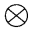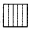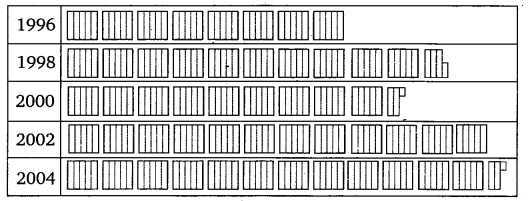# NCERT Solutions for Class 6 Maths Chapter 9 Data Handling Ex – 9.2

## Data Handling

Question 1.
Total number of animals in five villages are as follows:

Village A    :   80
Village B    :   120
Village C     :   90
Village D     :  40
Village E     :   60
Prepare a pictograph of these animals using one symbolto represent 10 animals and answer the following questions:
(a) How many symbols represent animals of village E?
(b) Which village has the maximum number of animals?
(c) Which village has more animals: village A or village C?

Solution:
The given data can be represented by a pictograph as given below:

(i)
6 symbols represent the animals of village E.
(ii) Village B has the maximum number of animals.
(iii) Village C has more animals than that of village A.

Question 2.
Total number of students of a school in different years is shown in the following table:

A. Prepare a pictograph of students using one symbol A to represent 100 students and answer the following questions:
(a) How many symbols represent total number of students in the year 2002?
(b) How many symbols represent total number of students for the year 1998?
B. Prepare another pictograph of students using any other symbol each representing 50 students. Which pictograph do you find more informative?

Solution:
The given data can be represented by a pictograph as given below:

(a)
6 symbols represent total number of students in the year 2002.
(b) 5 complete symbols and one incomplete symbol of 35 students
B. Let us prepare another pictograph of students using symboleach representing 50 students.